Next: Estimation of Variance Components Up: tr01cb1 Previous: Confounds

# Contrasts

This section demonstrates that the model equivalence result also holds when contrasts are used. That is, when contrasts are specified at the first level, only the contrasts of parameter estimates (copes), and their covariances, need to be used in the second level.

Contrasts are defined in the linear model in order to take linear combinations of the given parameters that form more meaningful or useful quantities. For instance, the difference between the responses to two different stimuli is often of greater interest than the individual responses, although it is easier to specify the design matrices,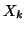, in terms of the individual stimuli.

Mathematically, each contrast is a column vector that represents how the old parameters,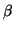, are combined to form new parameters,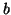. A collection of contrasts can be represented by a matrix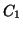, such that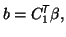where, for the time being, we assumeonly contains linearly independent contrasts.

When there are fewer contrasts specified than original parameters this implicitly defines a set of confounds. These confounds represent the remaining amount of signal modelled by the original parameters but not by the copes. It is shown in Theorem B (in the appendix) that a model with contrasts can be written as a model involving confounds but no contrasts. Using this theorem the problem can be reformulated into a confound problem for which model equivalence has already been established.

In terms of the second-level analysis, it is only the desired contrasts of parameter estimates (copes),, and their covariances,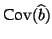, which are required. That is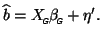Hence the introduction of contrasts does not affect the form of the model, but just substitutesandfor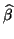and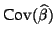respectively at the second-level.

In the above derivation, the only restriction is that the set of copes passed into the second level must not contain linear dependence. This is for precisely the same reasons that the regressors in any GLM must not be linearly dependent. Consequently, the set of contrasts specifying these copes at the first level must be linearly independent. This, however, does not prevent linearly dependent copes being estimated in the first level, but just that they cannot be passed into a second-level analysis all at once -- they would need to be combined into a linearly independent set first or tested in separate second-level analyses, if desired.

Consequently, all the previous results still hold when using contrasts.Next: Estimation of Variance Components Up: tr01cb1 Previous: Confounds
Christian Beckmann 2003-07-16